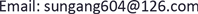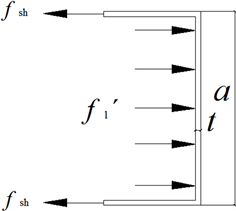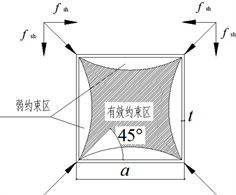﻿ 轴压下方形钢管混凝土短柱承载力的计算 The Calculation of Ultimate Strength for Axial-Loaded Square CFT Stub Columns

Hans Journal of Civil Engineering
Vol. 07  No. 06 ( 2018 ), Article ID: 27684 , 5 pages
10.12677/HJCE.2018.76112

The Calculation of Ultimate Strength for Axial-Loaded Square CFT Stub Columns

Gang Sun1, Xianrong Zhuo1, Guixin Ou2, Huayao Qiu1

1College of City Construction, Zhongkai University of Agriculture and Engineering, Guangzhou Guangdong

2Guangzhou Zhongda Vocational School of Science and Education, Guangzhou GuangdongReceived: Oct. 26th, 2018; accepted: Nov. 16th, 2018; published: Nov. 23rd, 2018ABSTRACT

The bearing mechanism of square concrete-filled steel tube stub column under axial load is analyzed. The effective confining coefficient and lateral compressive stress of square steel tube are determined based on the experimental data. Then, the calculated equations of square CFT subjected to axial load are proposed according to the typical confined concrete theory. Finally, 36 specimens are calculated by the proposed equations, and the calculated results are in accordance with the experimental and normative ones very well.

Keywords:Square CFT, Effective Confining Coefficient, Ultimate Strength

1仲恺农业工程学院城市建设学院，广东 广州

2广州市中大科教职业培训学校，广东 广州1. 引言

2. 方形钢管混凝土轴压短柱的计算

${N}_{c}={f}_{sl}{A}_{s}+{f}_{cc}{A}_{c}$ (1)

2.1. 方形钢管纵向应力fsl和环向应力fsh

${f}_{y}^{2}={f}_{sl}^{2}+{f}_{sh}^{2}$ (2)

${f}_{sh}=-0.21{f}_{y},\text{\hspace{0.17em}}{f}_{sl}=0.89{f}_{y}$ (3)

2.2. 核心混凝土轴心抗压强度fcc

2.2.1. 钢管有效侧向压应力的计算

${{f}^{\prime }}_{l}=2t{f}_{sh}/\left(a-2t\right)$ (4)Figure 1. Mechanic analysis of square steel tubeFigure 2. Confining mechanism of square CFT column

${K}_{\text{e1}}={A}_{e}/\left({A}_{c}+{A}_{e}\right)$ (5)

${k}_{\text{e}}={k}_{\text{e1}}{k}_{\text{e2}}$ (6)

${f}_{\text{l}}={k}_{\text{e}}{{f}^{\prime }}_{\text{l}}$ (7)

2.2.2. 核心混凝土轴心抗压强度fcc

${f}_{\text{cc}}={f}_{\text{c}}+k{f}_{\text{l}}$ (8)

3. 试验验证

Table 1. Comparison of ultimate strength between calculated results and experimental and normative ones on square CFT stub columns

4. 结语

1) 考虑钢管与混凝土之间的相互作用建立的该类构件的承载力计算公式，力学概念清晰，参数物理意义明确；

2) 用建议公式对试验试件进行承载力计算，计算结果与试验结果吻合良好。

3) 沿方形钢管壁中部设置约束拉杆，可以改善钢管对核心混凝土的约束效应。

The Calculation of Ultimate Strength for Axial-Loaded Square CFT Stub Columns[J]. 土木工程, 2018, 07(06): 938-942. https://doi.org/10.12677/HJCE.2018.76112

1. 1. EC4. (1996) Design of Steel and Concrete Structures. Part 1.1, General Rules for Building. DDEVV1994-1-1. British Standards Institute, London.

2. 2. 中华人民共和国住房和城乡建设部.钢管混凝土结构技术规范: GB50936-2014 [S]. 北京: 中国建筑工业出版社, 2014: 18-19.

3. 3. Richart, F.E. (1948) Reinforced Concrete Wall and Column Foots. ACI, 45, 97-127.

4. 4. Sakino, K., Nakahara, H., Morino, S. and Nishiyama, I. (2004) Behavior of Centrally Loaded Concrete-Filled Steel-Tube Short Columns. Journal of Structural Engineering, 130, No. 2.

5. 5. 张正国, 左明生. 方形钢管混凝土轴压短柱在短期一次静载下的基本性能研究[J]. 郑州工学院学报, 1985, 6(2): 19-32.

6. 6. Mander, J.B., Priestly, M.J.N. and Park, R. (1988) Theoretical Stress-Strain Model for Confined Concrete. Journal of Structural Engineering, 114, 1807-1826.